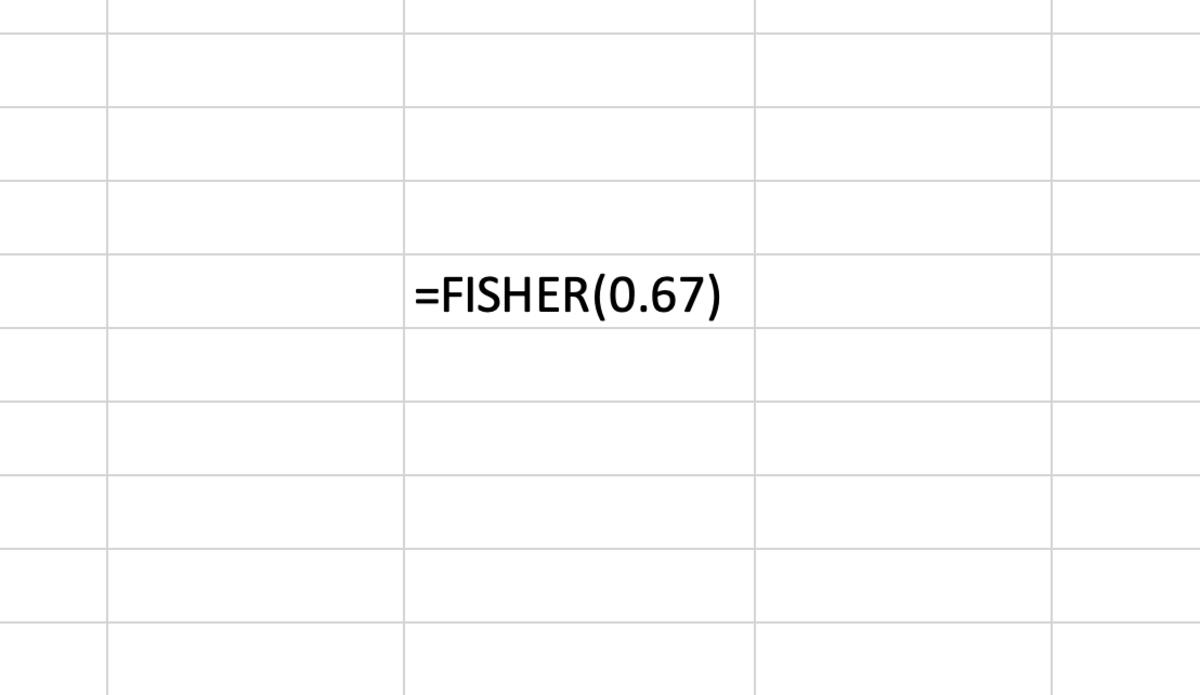# How to Use the FISHER Function in Excel for Mac

Joshua earned an MBA from USF and he writes mostly about software and technology.

## The Purpose of the FISHER Function

The FISHER function gives an Excel user the ability to complete a Fisher z transformation easily. The Fisher z transformation is a calculation that will transform Pearson’s correlation coefficient. The transformation from (r to zr) is used to calculate a confidence interval for specifically for the Pearson’s correlation coefficient.

The equation of the Fisher z transformation formula is z = ln((1+r) / (1-r)) / 2. You can also seen an alternate form of this equation illustrated below.

## The FISHER Function Syntax

The FISHER function needs to be inputted into a cell like a formula for it work. To manually add this function the following steps need to be taken:

1. A cell needs clicked type "=FISHER(".
2. After the open parenthesis the enter the correlation coefficient (r in the equation above)
3. Lastly, use closed parenthesis and enter the formula.

Below in bold the simple syntax of the FISHER function is shown in its entirety.

=FISHER(X)

X - The correlation coefficient. A number less than 1, but greater than or equal to zero. Using a number outside of this range or entering text into this function will cause and error. Note: This function only serves as a step to calculating a confidence interval.The FISHER function gives an Excel user the ability to complete a Fisher z transformation easily.

## Inserting the FISHER Function

The FISHER function can be inserted into a cell by first selecting a cell followed by clicking on the formula tab. Next, click on the more functions button and choose the FISHER function from the statistical function list.

Scroll to Continue

After the formula builder appears, add the correlation coefficient. Notice the formula builder provides tips for creating the function.

## Example

Entering a confidence coefficient of .67 results in .81074 as shown below.

Microsoft. (n.d.). FISHER function. Retrieved July 20, 2022, from https://support.microsoft.com/en-us/office/fisher-function-d656523c-5076-4f95-b87b-7741bf236c69

How to Use the COUNT Function in Excel

How to Use the AVERAGE Function in Excel

How to Use the ABS Function in Excel

How to Remove Errors in Excel With the IFERROR Function

How to Use the VLOOKUP Function in Excel

Converting Measurement Units: Using the CONVERT Function

To learn more about using functions in Excel I recommend The Excel 2019 Bible book. I have been using the Excel Bible for years to improve my understanding of all aspects of this Microsoft product.

This content is accurate and true to the best of the author’s knowledge and is not meant to substitute for formal and individualized advice from a qualified professional.